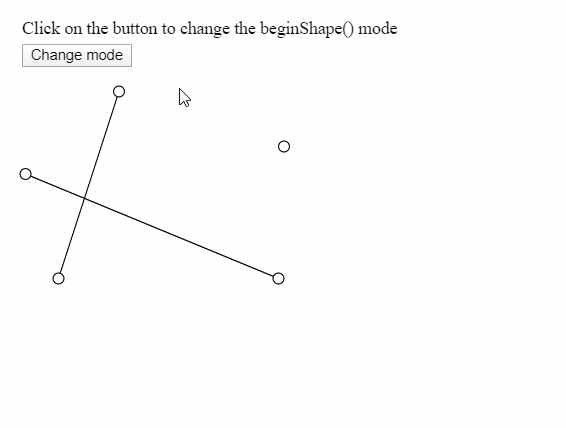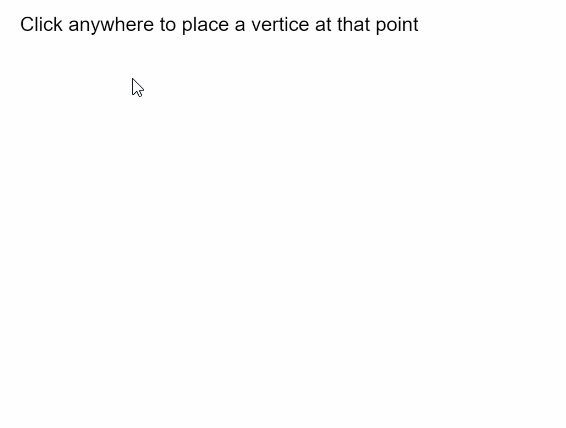# p5.js | beginShape() Function

• Difficulty Level : Basic
• Last Updated : 27 May, 2020

The beginShape() function in p5.js is used to draw more complex shapes. Specifying this function starts the recording of the vertices that would be used to draw the shape. The endShape() function must be called to end the recording and complete the shape.

After calling the beginShape() function, a series of vertices should be specified using the vertex() command. The shapes are outlined using the current stroke color and filled with the current fill color. There is an optional parameter that can be defined to use the kind of shape to be drawn.

The shapes drawn do not work with transformation functions such as translate(), rotate() and scale(). Also, it is not possible to use other shapes with beginShape().

Syntax:

`beginShape( [kind] )`

Parameters: This function accepts one parameter as mentioned above and described below:

• kind: It is a constant that can be used to change the kind of the shape drawn by this function. It can have the values of POINTS, LINES, TRIANGLES, TRIANGLE_FAN, TRIANGLE_STRIP, QUADS, QUAD_STRIP or TESS. It is an optional parameter.

The examples below illustrates the beginShape() function in p5.js:

Example 1:

 `let currMode;`` ` `function` `setup() {``  ``createCanvas(500, 300);``  ``textSize(18);`` ` `  ``let shapeModes = [LINES, TRIANGLES, TRIANGLE_FAN, TRIANGLE_STRIP, QUADS];``  ``let index = 0;``  ``currMode = shapeModes[index];`` ` `  ``let helpText = createP(``"Click on the button to change the beginShape() mode"``);``  ``helpText.position(20, 0);`` ` `  ``let closeBtn = createButton(``"Change mode"``);``  ``closeBtn.position(20, 40);``  ``closeBtn.mouseClicked(() => {``    ``if` `(index < shapeModes.length) index++;``    ``else` `index = 0;``    ``currMode = shapeModes[index];``  ``});``}`` ` `function` `draw() {``  ``clear();`` ` `  ``// Starting the shape using beginShape()``  ``beginShape(currMode);`` ` `  ``// Specifying all the vertices``  ``vertex(45, 245);``  ``vertex(100, 75);``  ``vertex(245, 245);``  ``vertex(15, 150);``  ``vertex(250, 125);`` ` `  ``// Ending the shape using endShape()``  ``endShape();`` ` `  ``// Points for demonstration``  ``circle(45, 245, 10);``  ``circle(100, 75, 10);``  ``circle(245, 245, 10);``  ``circle(15, 150, 10);``  ``circle(250, 125, 10);``}`

Output:Example 2:

 `let vertices = [];`` ` `function` `setup() {``  ``createCanvas(500, 300);``  ``textSize(18);`` ` `  ``text(``"Click anywhere to place a vertice at that point"``, 10, 20);``}`` ` `function` `mouseClicked() {``  ``// Update the vertices array with``  ``// current mouse position``  ``vertices.push({ x: mouseX, y: mouseY });`` ` `  ``clear();``  ``text(``"Click anywhere to place a vertice at that point"``, 10, 20);`` ` `  ``// Start the shape using beginShape()``  ``beginShape();`` ` `  ``// Use the vertices in the array``  ``// with the vertex() function``  ``for` `(let i = 0; i < vertices.length; i++)``    ``vertex(vertices[i].x, vertices[i].y);`` ` `  ``// End the shape using endShape()``  ``endShape();`` ` `  ``// Draw a circle at the last drawn vertice``  ``// for demonstration``  ``circle(mouseX, mouseY, 15);``}`

Output:Online editor: https://editor.p5js.org/

My Personal Notes arrow_drop_up